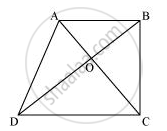Share

# Diagonals AC and BD of a trapezium ABCD with AB || DC intersect each other at O. Prove that ar (AOD) = ar (BOC). - Mathematics

Course

#### Question

Diagonals AC and BD of a trapezium ABCD with AB || DC intersect each other at O. Prove that ar (AOD) = ar (BOC).

#### SolutionIt can be observed that ΔDAC and ΔDBC lie on the same base DC and between the same parallels AB and CD.

∴ Area (ΔDAC) = Area (ΔDBC)

⇒ Area (ΔDAC) − Area (ΔDOC) = Area (ΔDBC) − Area (ΔDOC)

⇒ Area (ΔAOD) = Area (ΔBOC)

Is there an error in this question or solution?